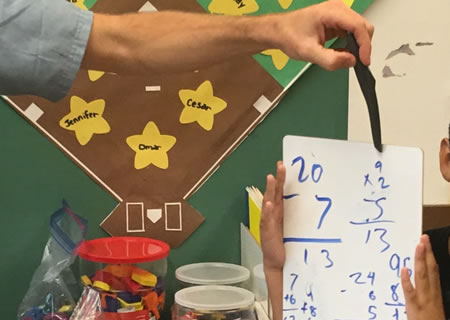Home Free Lessons Target Number##### Description
• Author:
• Posted:
• Topics:
, , , , , ,
• Type:
• Keywords:

Math Concepts: Arithmetic, equivalencies
Equipment: pencil & paper
Grades: K, 1, 2, 3, 4, 5
Common Core: Variable, but especially OA, i.e., 1.OA.C.5, 2.OA.B.2, 3.OA.C.7
Time: 5 – 15 minutes

You know the answer. What’s the question?

#### Why We Love Target Number

This is a quick check-in that adjusts to the abilities of each student, allows for creativity and arithmetic practice together, and is a lot of fun.
A perfect warm-up.

#### The Activity

The teacher writes a “target” number on the board. The students try to write down as many different number sentences that have that the target number as the answer. Then students share their favorite answers. For younger students, drawing different pictures or arrangements of ways to see/understand that number is an ok alternative.

Example

The teacher write 7 on the board, and let’s kids write on their own paper for about a minute, then asks students to share what they found. Students raise their hands to volunteer solutions while the teacher writes them on the board.
These equations may go from simple equations like 6 + 1 = 7 to the more complex (4 x 3) – 5 = 7.
The great thing is, anyone can start, but the sky is the limit!

#### Questions

Don’t do these on day 1, but if you want to go deeper with this activity, here are some interesting questions to pursue.

• If we only add 2 numbers, how many answers can we find?
• What if we add 3 numbers, or 4 numbers?
• What about any number of numbers?
• What if we only subtract, or only multiply, or only divide?
• What’s the longest number sequence you can find that hits the target number?
• Can you hit the target number if you only use a single number, such as the number 4, in your equation?

#### Tips for the Classroom

1. Don’t praise answers with many steps as “smart.” This activity gives everyone a chance to contribute and be valued. You can describe those answers as “long,” or as having many parts.
2. If answers are wrong or unclear, you can take the opportunity to do the arithmetic with the class. On the other hand, if a student uses terms (like square root) that the class isn’t ready for yet, you can write down their answer but move on to other solutions.
3. One opportunity this lesson gives you is the chance to emphasize equivalency.
If one student knows that 6+1 = 7, and someone else knows that (3 x 4) – 5 = 7, then that means that 6 + 1 = (3 x 4) – 5.
It’s nice to underline the point that there are many ways to equal 7, and that these ways are all equal to each other.
4. Let’s say someone says that 7 = 5 + 3. Rather than just saying “wrong,” say that 5 + 3 gets us close to 7, but we need to do something else to get all the way there, then challenge students to find what still needs to be done.
If someone can explain that 5 + 3 is 8, and so you need to take 1 away, you have the number sentence 7 = 5 + 3 – 1.
This is both more sophisticated and accepts the original students wrong answer as a path toward a better, accurate answer, rather than a dead end.